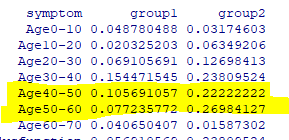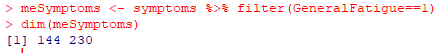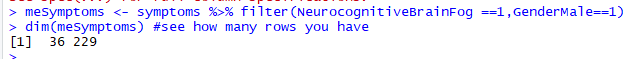# Building a model of Symptoms

We will continue onwards from my last post . Our first step is to determine how many clusters (groupings) of the symptoms that our current data supports.

We do this by trying every size up to 25 and then inspect the chart. The first part is pretty standard R code.

symptoms <-read_csv(“http://lassesen.com/ubiome/SymptomsObservations.csv”) scaled=scale(symptoms) par(mfrow = c(1, 1)) wss <-0 for (i in 1:25) { km.out <- kmeans(scaled, centers = i, nstart = 20, iter.max = 50) wss[i] <- km.out\$tot.withinss }

We then go and drop this data on a plot:

plot(1:25, wss, type = “b”, main=”Ability to decompose symptoms”, xlab = “Number of Clusters”, ylab = “Within groups sum of squares”)

The chart indicate that we likely only have two clusters that are distinct. While our hope was to be able to better cluster people by their symptoms — there is just not enough data yet.

We can look at just 2 clusters with this code

``` km.out <- kmeans(scaled, centers = 2,nstart = 20, iter.max = 50) km.out ```

This gives us a list(km.out\$cluster) showing which symptom belongs to which group. We then compute the average (mean) for each group and each symptom. We then do some manipulation to change the structure of the table so we get symptom, average in Group1, average in Group 2.

symptoms\$cluster=km.out\$cluster
group1 <-aggregate(symptoms, by=list(symptoms\$cluster),mean) %>% gather(symptom,group,-cluster) %>% filter(cluster==1)
group2 <-aggregate(symptoms, by=list(symptoms\$cluster),mean) %>% gather(symptom,group,-cluster) %>% filter(cluster==2)
colnames(group2) = c(“c”,”symptom”,”group2″) colnames(group1) = c(“c”,”symptom”,”group1″) report<-merge(group1,group2,by=”symptom”) %>% select(symptom,group1,group2)
reportWe see that one group has more people in the 40-60 range.

We can then sort the symptoms by the differences between group:

report %>% arrange(desc(group2-group1))We see that one group has a high rate of neurological issues and the other does not.

For symptoms that do not vary much between the groups we see a lot of official diagnosis that are not ME/CFS like –

## Building a model for ME/CFS only

The above was done for ALL samples uploaded — a mixed bag. As see, we could classify by symptoms into a ME/CFS group (Group 2) and a non ME/CFS group (Group 1).

With the above R-Scripts we can apply it to a subset that we define by selecting one or more symptoms as being required. For ME/CFS: GeneralFatigue.This gives us 144 observations

And the same code as above

Again the chart suggests that two clusters is what the data supports.

Create the report, as above:

And now the symptoms that MOST separate the groups. We see that one group was various symptoms — having any would drop them into Group 1.

Looking at what least separate we see a lot of symptoms with no observations that also had general fatigue. Many of these symptoms are associated with ME/CFS — This implies that the picking of
general fatigue alone, was likely a bad choice.

## BOTTOM LINE

This post intent was NOT to discover an interesting fact, but to show how you can explore the data (and hopefully discover an interesting fact that you will share).

To make your life easier, I have the R code needed below to do your investigations.

library(tidyverse)
symptoms <-read_csv(“http://lassesen.com/ubiome/SymptomsObservations.csv”) meSymptoms <- symptoms %>% filter([YOUR ITEM 1]==1,[YOUR ITEM 2]==1)
dim(meSymptoms) #see how many rows you have

par(mfrow = c(1, 1))
wss <-0
for (i in 1:25) {
# Fit the model: km.out
km.out <- kmeans(meSymptoms, centers = i, nstart = 20, iter.max = 50)
# Save the within cluster sum of squares
wss[i] <- km.out\$tot.withinss
}
plot(1:25, wss, type = “b”, main=”Ability to decompose ME symptoms”,
xlab = “Number of Clusters”,
ylab = “Within groups sum of squares”)

meSymptoms\$cluster=km.out\$cluster
group1 <-aggregate(meSymptoms, by=list(meSymptoms\$cluster),mean) %>% gather(symptom,group,-cluster) %>% filter(cluster==1)
group2 <-aggregate(meSymptoms, by=list(meSymptoms\$cluster),mean) %>% gather(symptom,group,-cluster) %>% filter(cluster==2)
colnames(group2) = c(“c”,”symptom”,”group2″)
colnames(group1) = c(“c”,”symptom”,”group1″)
report<-merge(group1,group2,by=”symptom”) %>% select(symptom,group1,group2)
report %>% arrange(desc(abs(group2-group1))) #most different
report %>% arrange(abs(group2-group1)) #least different

### Caution

When the number of observations go low, you may get no meaningful results. Example:Just 36 rows

We expect to see a big drop initially and then a clear “elbow” in the curve. There was none appearing with the above.